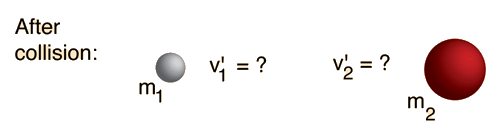In the general case of a one-dimensional collision between two masses, one cannot anticipate how much kinetic energy will be lost in the collision. Therefore, the velocities of the two masses after the collision are not completely determined by their velocities before the collision. However, conservation of momentum must be satisfied, so that if the velocity of one of the particles after the collision is specified, the other is determined.Initial conditions:
 m1=kg, v1=m/s, pi=kg m/s m2=kg, v2=m/s, KEi=J
Enter values for masses and velocities above. Then specify one final velocity below.Final velocities(specify one, the other will be calculated): v'1= m/s, v'2= m/s
 Final momentum = pf=kg m/s, Final kinetic energy = KEf = J
 For a perfectly elastic collision, the values would have been: v'1 = m/s, v'2 = m/s

For ordinary objects, the final kinetic energy will be less than the initial value. The only way you can get an increase in kinetic energy is if there is some kind of energy release triggered by the impact. Was one of your objects a hand grenade?

### Examine the case where the masses stick together.

Index

Collision concepts

Elastic collisions